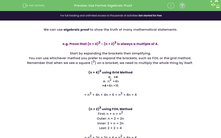# Use Formal Algebraic Proof

In this worksheet, students will learn how to express mathematical proofs and the features of formal algebraic proof which students need to be familiar with using.Key stage:  KS 4

Year:  GCSE

GCSE Subjects:   Maths

GCSE Boards:   Pearson Edexcel, OCR, Eduqas, AQA,

Curriculum topic:   Algebra

Curriculum subtopic:   Notation, Vocabulary and Manipulation Algebraic Expressions

Popular topics:   Algebra worksheets

Difficulty level:#### Worksheet Overview

We can use algebraic proof to show the truth of many mathematical statements.

e.g. Prove that (n + 4)2 - (n + 2)2 is always a multiple of 4.

Start by expanding the brackets then simplifying.

You can use whichever method you prefer to expand the brackets, such as FOIL or the grid method.

Remember that when we see a square (2) on a bracket, we need to multiply the whole thing by itself.

(n + 4)2 using Grid Method

 n +4 n n2 +4n +4 +4n +16

= n2 + 4n + 4n + 6 = n2 + 8n + 6

(n + 2)2 using FOIL Method

First: n × n = n2

Outer: n × 2 = 2n

Inner: 2 × n = 2n

Last: 2 × 2 = 4

= n2 + 2n + 2n + 4 = n2 + 4n + 4

n2 + 4n + 4n +16 - (n2 +2n + 2n + 4)

= n2 + 4n + 4n + 16 - n2 - 2n - 2n - 4

= 4n + 12

Then we need to factorise to show that 4 is a factor and that the expression is a multiple of 4:

4(n + 3)

We use a set of expression to show different kinds of numbers.

These help us with writing algebraic proofs:

 2n an even number 2n + 1 an odd number n, n+1, n+2 consecutive numbers 2n, 2n+2, 2n+4 consecutive even numbers 2n+1, 2n+3, 2n+5 consecutive odd numbers 6n multiple of 6 5n multiple of 5

Have a look at this question now.

e.g. Prove that the sum of any three consecutive even numbers is always a multiple of 6.

We are going to show that adding three consecutive even numbers (2n, 2n+2, 2n+4) gives a multiple of 6:

2n + 2n + 2 + 2n + 4

Simplify:

6n + 6

Factorise to show 6 is a factor:

6(n + 1)

In this activity, we will learn more about how to express mathematical proofs and the features of formal algebraic proof which you need to be familiar with using.

You may want to have a pen and paper handy to record your working, as some of these proofs can become quite complex.

### What is EdPlace?

We're your National Curriculum aligned online education content provider helping each child succeed in English, maths and science from year 1 to GCSE. With an EdPlace account you’ll be able to track and measure progress, helping each child achieve their best. We build confidence and attainment by personalising each child’s learning at a level that suits them.

Get started